The Decagons ClipArt gallery offers 13 illustrations of geometric shapes that are composed of 10 sides, and usually refers to regular decagons, where all sides are equal and all angles are equal to 144 degrees.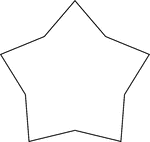### Concave Equilateral Decagon

A 5-point star made from a non-regular concave decagon in which all sides are equal in length.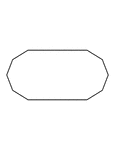### Irregular Convex Decagon

Illustration of an irregular convex decagon. This polygon has some symmetry.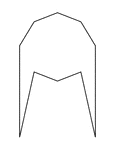### Irregular Convex Decagon

Illustration of an irregular convex decagon. This polygon has some symmetry.### Irregular Convex Decagon

Illustration of an irregular convex decagon. This polygon has some symmetry.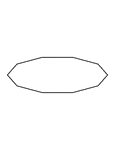### Irregular Convex Decagon

Illustration of an irregular convex decagon. This polygon has some symmetry.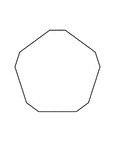### Irregular Convex Decagon

Illustration of an irregular convex decagon. This polygon has some symmetry.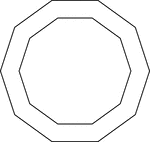### 2 Concentric Decagons

Illustration of 2 regular concentric decagons.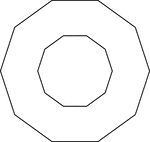### 2 Concentric Decagons

Illustration of 2 regular concentric decagons.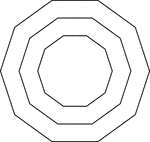### 3 Concentric Decagons

Illustration of 3 regular concentric decagons that are equally spaced.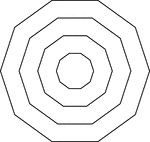### 4 Concentric Decagons

Illustration of 4 regular concentric evenly spaced decagons.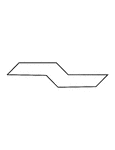### Irregular Concave Octagon

Illustration of an irregular concave octagon. This polygon has some symmetry.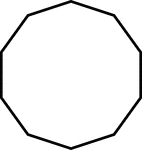### 10-sided Polygon

Polygon consisting of 10 sides